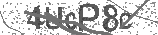﻿ Introduction to Statistics | Safe Videos for Kids
Welcome

# Introduction to Statistics

Thanks! Share it with your friends!

URL

You disliked this video. Thanks for the feedback!

Sorry, only registred users can create playlists.URLChannel: The Organic Chemistry Tutor
Categories: Statistics & Probability   |   MathFind Related Videos  added
22 Views## Description

This video tutorial provides a basic introduction into statistics. It explains how to find the mean, median, mode, and range of a data set. It also explains how to find the interquartile range, quartiles, percentiles as well as any outliers. The full version of this video which can be found on my patreon page also mentions how to construct box and whisker plots, histograms, frequency tables, frequency distribution tables, dot plots, and stem and leaf plots. It also covers relative frequency and cumulative relative frequency as well as how to use it to determine the value that a corresponds to a certain percentile. Finally, this video also discusses skewness - it explains which distribution is symmetric and which is skewed to the right (positive skew) and which is skewed to the left (negative skew).

Full 1 Hour Video:

How To Calculate The Mean, Median, Mode, Range, Variance & Standard Deviation Using Excel:Be the first to comment